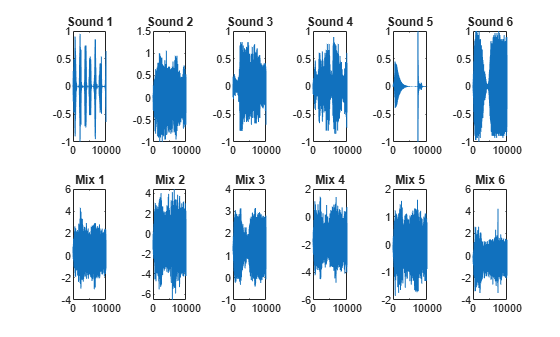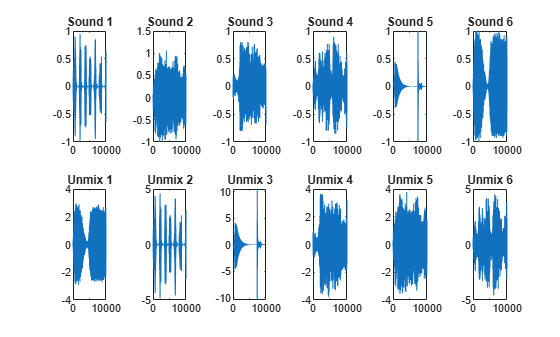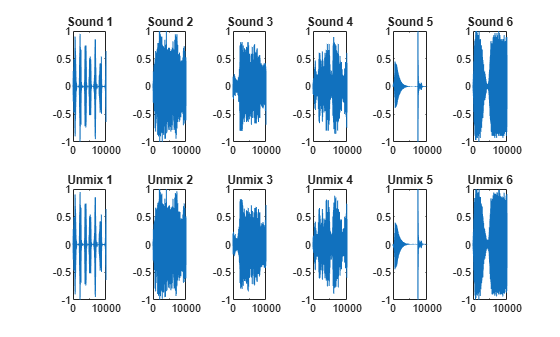# Extract Mixed Signals

This example shows how to use rica to disentangle mixed audio signals. You can use rica to perform independent component analysis (ICA) when prewhitening is included as a preprocessing step. The ICA model isHere,is a-by-1 vector of mixed signals,is a-by-1 vector of offset values,is a-by-mixing matrix, andis a-by-1 vector of original signals. Suppose first thatis a square matrix. If you knowand, you can recover an original signalfrom the data:Using the rica function, you can perform this recovery even without knowing the mixing matrixor the mean. Given a set of several observations,, ..., rica extracts the original signals,, ....

Load a set of six audio files, which ship with MATLAB®. Trim each file to 10,000 samples.

files = {'chirp.mat' 'gong.mat' 'handel.mat' 'laughter.mat' 'splat.mat' 'train.mat'}; S = zeros(10000,6); for i = 1:6 test = load(files{i}); y = test.y(1:10000,1); S(:,i) = y; end 

### Mix Signals

Mix the signals together by using a random mixing matrix and add a random offset.

rng default % For reproducibility mixdata = S*randn(6) + randn(1,6); 

To listen to the original sounds, execute this code:

 for i = 1:6 disp(i); sound(S(:,i)); pause; end

To listen to the mixed sounds, execute this code:

 for i = 1:6 disp(i); sound(mixdata(:,i)); pause; end

Plot the signals.

figure for i = 1:6 subplot(2,6,i) plot(S(:,i)) title(['Sound ',num2str(i)]) subplot(2,6,i+6) plot(mixdata(:,i)) title(['Mix ',num2str(i)]) endThe original signals have clear structure. The mixed signals have much less structure.

### Prewhiten Mixed Signals

To separate the signals effectively, "prewhiten" the signals by using the prewhiten function that appears at the end of this example. This function transforms mixdata so that it has zero mean and identity covariance.

The idea is the following. Ifis a zero-mean source with statistically independent components, thenThen the mean and covariance ofareSuppose that you knowand. In practice, you would estimate these quantities from the sample mean and covariance of the columns of. You can solve forin terms ofbyThe latter equation holds even whenis not a square invertible matrix.

Suppose thatis a-by-matrix of left eigenvectors of the positive semidefinite matrix, andis the-by-matrix of eigenvalues. ThenThenThere are many mixing matricesthat satisfy this last equation. Ifis a-by-orthonormal matrix, thenSubstituting into the equation for,is the prewhitened data. rica computes the unknown matrixunder the assumption that the components ofare as independent as possible.

mixdata = prewhiten(mixdata); 

### Separate All Signals

A super-Gaussian source has a sharp peak near zero, such as a histogram of sound 1 shows.

figure histogram(S(:,1))Perform Reconstruction ICA while asking for six features. Indicate that each source is super-Gaussian.

q = 6; Mdl = rica(mixdata,q,'NonGaussianityIndicator',ones(6,1)); 

Extract the features. If the unmixing procedure is successful, the features are proportional to the original signals.

unmixed = transform(Mdl,mixdata); 

### Compare Unmixed Signals To Original Signals

Plot the original and unmixed signals.

figure for i = 1:6 subplot(2,6,i) plot(S(:,i)) title(['Sound ',num2str(i)]) subplot(2,6,i+6) plot(unmixed(:,i)) title(['Unmix ',num2str(i)]) endThe order of the unmixed signals is different than the original order. Reorder the columns so that the unmixed signals match the corresponding original signals. Scale the unmixed signals to have the same norms as the corresponding original signals. (rica cannot identify the scale of the original signals because any scale can lead to the same signal mixture.)

unmixed = unmixed(:,[2,5,4,6,3,1]); for i = 1:6 unmixed(:,i) = unmixed(:,i)/norm(unmixed(:,i))*norm(S(:,i)); end 

Plot the original and unmixed signals.

figure for i = 1:6 subplot(2,6,i) plot(S(:,i)) ylim([-1,1]) title(['Sound ',num2str(i)]) subplot(2,6,i+6) plot(unmixed(:,i)) ylim([-1,1]) title(['Unmix ',num2str(i)]) endThe unmixed signals look similar to the original signals. To listen to the unmixed sounds, execute this code.

 for i = 1:6 disp(i); sound(unmixed(:,i)); pause; end

Here is the code for the prewhiten function.

function Z = prewhiten(X) % X = N-by-P matrix for N observations and P predictors % Z = N-by-P prewhitened matrix % 1. Size of X. [N,P] = size(X); assert(N >= P); % 2. SVD of covariance of X. We could also use svd(X) to proceed but N % can be large and so we sacrifice some accuracy for speed. [U,Sig] = svd(cov(X)); Sig = diag(Sig); Sig = Sig(:)'; % 3. Figure out which values of Sig are non-zero. tol = eps(class(X)); idx = (Sig > max(Sig)*tol); assert(~all(idx == 0)); % 4. Get the non-zero elements of Sig and corresponding columns of U. Sig = Sig(idx); U = U(:,idx); % 5. Compute prewhitened data. mu = mean(X,1); Z = bsxfun(@minus,X,mu); Z = bsxfun(@times,Z*U,1./sqrt(Sig)); end